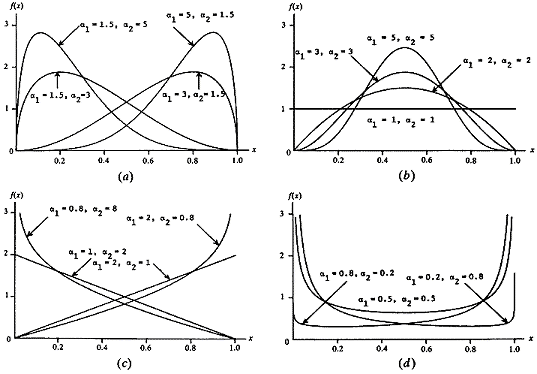# Expected Random Sum Above 1

Probability Level 5Starting with $S= 0$, you choose a number between $0$ and $1$ at random and add it to $S$. If $S< 1$, you repeat and choose a number between $0$ and $1$ at random and add it to $S$. If $S \geq 1$, you stop.

If the expected value of $S$ when you stop is denoted by $E[S]$, what is the integer closest to $\frac { 200 \times E[S] }{ e^2 } ?$

×